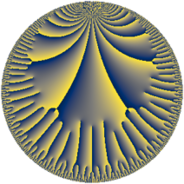# Properties

 Label 24.22.dLevel $24$ Weight $22$ Character orbit 24.d Rep. character $\chi_{24}(13,\cdot)$ Character field $\Q$ Dimension $42$ Newform subspaces $1$ Sturm bound $88$ Trace bound $0$

# Related objects

## Defining parameters

 Level: $$N$$ $$=$$ $$24 = 2^{3} \cdot 3$$ Weight: $$k$$ $$=$$ $$22$$ Character orbit: $$[\chi]$$ $$=$$ 24.d (of order $$2$$ and degree $$1$$) Character conductor: $$\operatorname{cond}(\chi)$$ $$=$$ $$8$$ Character field: $$\Q$$ Newform subspaces: $$1$$ Sturm bound: $$88$$ Trace bound: $$0$$

## Dimensions

The following table gives the dimensions of various subspaces of $$M_{22}(24, [\chi])$$.

Total New Old
Modular forms 86 42 44
Cusp forms 82 42 40
Eisenstein series 4 0 4

## Trace form

 $$42 q - 574 q^{2} + 392288 q^{4} - 44759142 q^{6} - 1129900996 q^{7} - 2157413692 q^{8} - 146444944842 q^{9} + O(q^{10})$$ $$42 q - 574 q^{2} + 392288 q^{4} - 44759142 q^{6} - 1129900996 q^{7} - 2157413692 q^{8} - 146444944842 q^{9} + 31553345052 q^{10} - 274707993996 q^{12} + 1565450468972 q^{14} + 2306601562500 q^{15} - 3863799224840 q^{16} - 3481428994004 q^{17} + 2001414246174 q^{18} + 72162740641304 q^{20} - 372001656147968 q^{22} - 174381110476664 q^{23} - 137569089593988 q^{24} - 3726960758912246 q^{25} + 1821164548445192 q^{26} + 6111351944921536 q^{28} - 3695078269676844 q^{30} + 13907033543056644 q^{31} + 20291511717782536 q^{32} - 26951524530024580 q^{34} - 1367823679099488 q^{36} + 152044668976276888 q^{38} - 65123248681532808 q^{39} + 48530287268994440 q^{40} + 85901867185267604 q^{41} + 164202494061337308 q^{42} - 583729140892614912 q^{44} - 425500141029899224 q^{46} - 1351800616447161624 q^{47} - 375977886927896448 q^{48} + 3990677511431283522 q^{49} + 3155333405061952034 q^{50} + 1393522695028139216 q^{52} + 156065478127743942 q^{54} - 89167753774449664 q^{55} - 1787796305200178632 q^{56} - 2098638974607729624 q^{57} - 7254382453095841940 q^{58} - 6972548942016787968 q^{60} - 15146072229522685484 q^{62} + 3939721167527163396 q^{63} + 34964359458467535488 q^{64} - 12603807792794356144 q^{65} - 43379940017819256192 q^{66} + 41027785784298520512 q^{68} + 17400423406741356136 q^{70} - 101755763943373821288 q^{71} + 7522436407769418492 q^{72} + 7031925768882255172 q^{73} + 156212595524575515440 q^{74} + 120422011169859891760 q^{76} + 29908411169744718936 q^{78} + 260653560486670585748 q^{79} - 168480498883332591744 q^{80} + 510621949280391009642 q^{81} - 354029829364220187068 q^{82} - 220644844170169622760 q^{84} + 49699114310265976040 q^{86} - 298108097029722826188 q^{87} + 184453501508512377472 q^{88} - 493168983092689682020 q^{89} - 110019711326684133852 q^{90} + 461231995073255535744 q^{92} + 690723142138680165384 q^{94} + 2751494378446039662064 q^{95} + 1069806011881439468808 q^{96} - 227026944571268558932 q^{97} + 1371753640376248773498 q^{98} + O(q^{100})$$

## Decomposition of $$S_{22}^{\mathrm{new}}(24, [\chi])$$ into newform subspaces

Label Dim $A$ Field CM Traces $q$-expansion
$a_{2}$ $a_{3}$ $a_{5}$ $a_{7}$
24.22.d.a $42$ $67.075$ None $$-574$$ $$0$$ $$0$$ $$-1129900996$$

## Decomposition of $$S_{22}^{\mathrm{old}}(24, [\chi])$$ into lower level spaces

$$S_{22}^{\mathrm{old}}(24, [\chi]) \cong$$ $$S_{22}^{\mathrm{new}}(8, [\chi])$$$$^{\oplus 2}$$